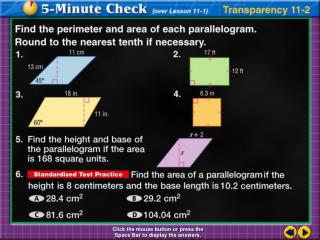DownloadDownload PresentationTransparency 2

Transparency 2

Télécharger la présentationTransparency 2

- - - - - - - - - - - - - - - - - - - - - - - - - - - E N D - - - - - - - - - - - - - - - - - - - - - - - - - - -
Presentation Transcript

1. Transparency 2 Click the mouse button or press the Space Bar to display the answers.

2. Transparency 2a

3. Lesson 11.2 Key Notes • Area of a Triangle: • Area of a Trapezoid: • Area of a Rhombus: • Postulate 11.1 Congruent figures have equal areas

4. Find the area of quadrilateral ABCD if AC 35, BF 18, and DE 10. The area of the quadrilateral is equal to the sum of the areas of Example 2-1a Area formula Substitution Simplify. Answer: The area of ABCD is 490 square units.

6. Bases:Since and are horizontal, find their length by subtracting the x-coordinates of their endpoints. Example 2-2a Find the area of trapezoid RSTU with vertices R(4, 2), S(6, –1), T(–2, –1), and U(–1, 2).

7. Example 2-2a Height: Because the bases are horizontal segments, the distance between them can be measured on a vertical line. That is, subtract the y-coordinates. Area: Area of a trapezoid Simplify. Answer: The area of trapezoid RSTU is 19.5 square units.

8. Example 2-2b Find the area of trapezoid WXYZ with vertices W(–3, 0), X(1, 0), Y(2, –3), and Z(–5, –3). Answer:

9. Example 2-3a Find the area of rhombus MNPR with vertices at M(0, 1), N(4, 2), P(3, –2), and R(–1, –3). Explore To find the area of the rhombus, we need to know the lengths of each diagonal. Plan Use coordinate geometry to find the length of each diagonal. Use the formula to find the area of rhombus MNPR.

10. Solve Use the Distance Formula to find . Use the Distance Formula to find . Example 2-3a

11. Example 2-3a Area of a rhombus Examine The area of rhombus MNPR is 15 square units. Answer: 15 square units

12. Answer: Example 2-3b Find the area of rhombus ABCD with vertices A(–3, 3), B(2, 2), C(3, –3), and D(–2, –2).

13. Rhombus RSTU has an area of 64 square inches. Find if inches. Answer:US is 16 inches long. Example 2-4a Use the formula for the area of a rhombus and solve for d2.

14. Example 2-4b Trapezoid DEFGhas an area of 120 square feet. Find the height of DEFG. Use the formula for the area of a trapezoid and solve for h. Answer: The height of trapezoid DEFG is 8 feet.

15. a. Rhombus ABCD has an area of 81 square centimeters. Find BD if centimeters. Example 2-4c Answer: 27 cm b. Trapezoid QRST has an area of 210 square yards. Find the height of QRST. Answer: 6 yd

16. First, find the area of one trapezoid. From Postulate 11.1, the area of each trapezoid is the same. So, the area of each trapezoid is or 9 square feet. Example 2-5a STAINED GLASS This stained glass window is composed of 8 congruent trapezoidal shapes. The total area of the design is 72 square feet. Each trapezoid has bases of 3 and 6 feet. Find the height of each trapezoid. Next, use the area formula to find the height of each trapezoid.

17. Example 2-5a Area of a trapezoid Substitution Add. Multiply. Divide each side by 4.5. Answer: Each trapezoid has a height of 2 feet.

18. Example 2-5b INTERIOR DESIGNThis window hanging is composed of 12 congruent trapezoidal shapes. The total area of the design is 216 square inches. Each trapezoid has bases of 4 and 8 inches. Find the height of each trapezoid. Answer: 3 in.

19. HW #1: p. 598 10-22 evens, 27-30 all (11 problems)HW #2: p. 606 14-34 evens (11 problems)